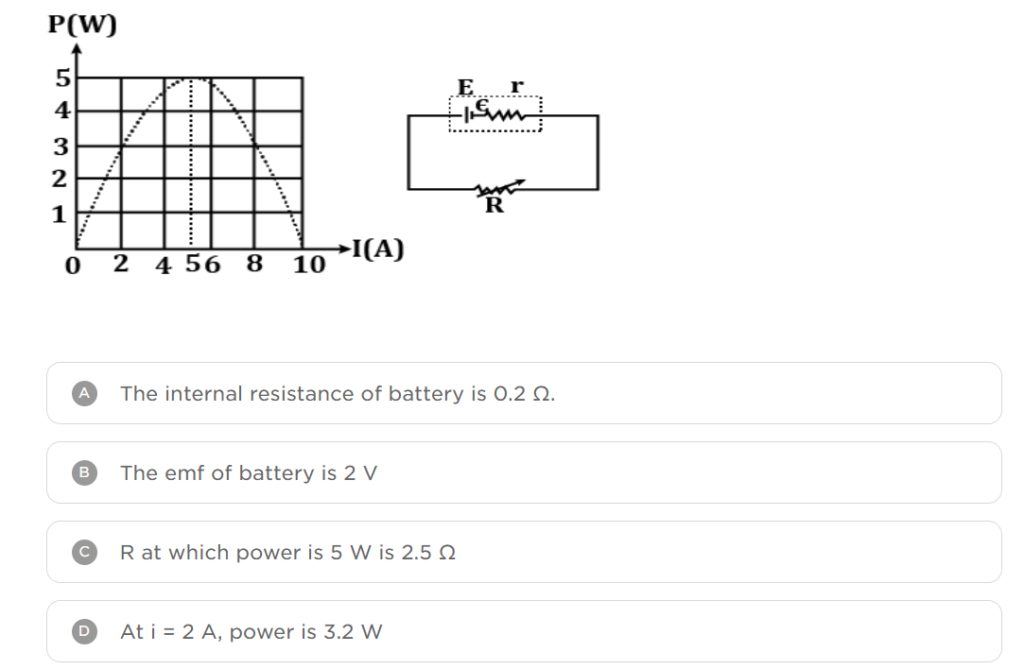Online Tution   »   Important Question   »   Net Power Dissipated

# What is Net power dissipated?

## Net Power Dissipated: Definition

The net power dissipated in an AC circuit with maximum amplitude current flowing through a resistance is less than the power generated by a steady DC current of magnitude Im passing through the same resistance. The procedure by which electronic or electrical equipment generates heat (power losses or wastage) as an unwanted offshoot of its primary activity is defined as power dissipation. Net Power dissipation is a major consideration in computer architecture, as it is with central processing units.

## Net Power Dissipated: Concept

Net Power dissipation in resistors is thought to be a natural occurrence. The truth remains that any resistors in a circuit with a voltage drop across them will dissipate electrical energy. Furthermore, because electrical power is converted into heat energy, all resistors have a (power) rating. A resistor’s power rating is also a categorization that specifies how much power it can dissipate before reaching critical failure. As you may know, power is measured in Watts (W), and the formula for power is

Power = Current x Voltage

 =>       P = I . V

According to the rules of physics, if the voltage (E) is increased, the current (I) will increase as well, and the power dissipation of a resistor will increase as well. However, if the resistor’s value is increased, current is reduced, and the resistor’s power dissipation is reduced as well. This relationship is based on Ohm’s law, which states that current is defined as

Current = Voltage x Resistance

 =>       I = V . R

## Net Power Dissipated: How to Calculate

Power dissipation is a measurement metric in the world of electronics that quantifies the release of heat within a circuit owing to inefficiencies. To put it another way, net power dissipation is a measurement of how much power is turned into heat in a circuit. As I previously stated, each resistor has a power rating, which allows designers to determine whether or not a specific resistor will match their design requirements within a circuit. So, let’s look at how to calculate this crucial design parameter in more detail.

According to the Ohm’s law,

Voltage = Current) × Resistance

 =>     V = I . R

Current = Voltage ÷ Resistance

 =>     I = V / R

Power = Current × Voltage

 =>     P = I . V

Hence, calculation of the power dissipation by the resistor can be done using the following formula:

Net Power dissipated = (Current)2 × Resistance

 =>     P = I2 . R

or, we can also use the formula:

Net Power Dissipated = (Voltage)2 ÷ Resistance)

 =>     P = V2 / R

## Net Power Dissipated: Positive or Negative

A passive component that uses energy, such as a light bulb or an appliance, will have positive power dissipation, whereas an active ingredient, such as a power source or batteries, will still have negative power dissipation.

In General, power dissipation is not considered very good, but there are specific situations where heat dissipation is beneficial. Consider electric heaters that make use of Nichrome resistance wire. Because of its cost-effectiveness, resistance to electron flow, strength, flexibility, oxidation resistance, and stability at high temperatures, nichrome is a one-of-a-kind heating element. Incandescent light bulbs, which are used as cost-effective heaters, are another example where heat dissipation is beneficial. Under most conditions, heat dissipation is undesirable; but, on the few occasions when it is, efforts to control rather than moderate heat dissipation will be made.

## Figure Shows the Net Power DissipatedAnswer is D

Related Post:

## Net Power Dissipated: FAQs

Ques. Is energy dissipated negative?

Ans. When U0 is small, energy is removed from the resistor and used to cool the environment, indicating negative energy dissipation. Thermal equilibrium is represented by the voltage, which is a thermodynamic quantity.

Ques. Is power dissipated always positive?

Ans. In a resistive circuit, current and voltage are in phase. For this resistive circuit, the power waveform is always positive, never negative. This means that, unlike reactive loads, power is always absorbed by the resistive load and never restored to the source.

Ques. What is net power dissipation?

Ans. The process by which an electronic or electrical device produces heat (energy loss or wastage) as an unwanted offshoot of its primary activity is defined as net power dissipation.

Ques. What is the formula for the net power dissipated in a resistor?

Ans. Because all three are known, the power wasted by each resistor may be calculated using any of the equations relating power to current, voltage, and resistance. Because each resistor receives full power, we’ll use P = V2 . R and P = V2 R.

Ques. Does power dissipated equal power supplied?

Ans. The power supplied by the voltage source and that wasted by the resistor are same in a basic circuit with one voltage source and a single resistor. In more intricate circuits, P might refer to the power dissipated by a single device rather than the circuit’s total power.

Sharing is caring!

Thank You, Your details have been submitted we will get back to you.